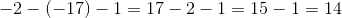Example Questions

Example Question #45 : Integers

How many integers are there between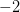and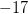(not includingor)?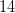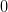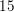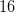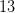Explanation:

Since we are not includingorin the count, the number of integers is given by the equation: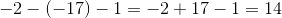If negative numbers are difficult for you to understand you can think of it this way. Since both values are negative and we are looking for the amount of integers between them we can solve the problem by subtracting 2 from 17 and then another one since we don't want to include the endpoints.